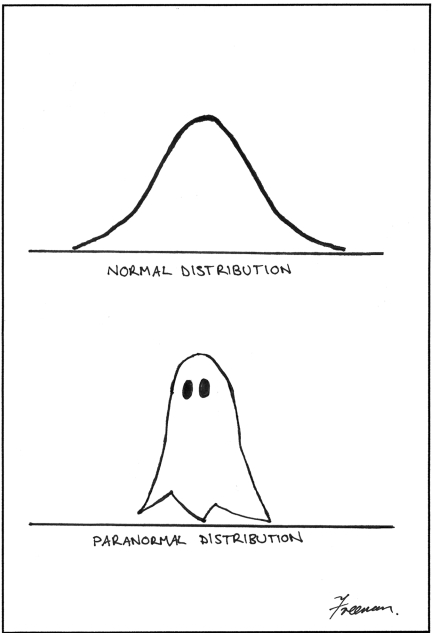## Exercise 1

Consider two random variables $$X$$ and $$Y$$ with

• $$\sigma_{X} = 2$$
• $$\sigma_{Y} = 3$$
• $$\text{Var}[4X-5Y] = 481$$.

What is the correlation between $$X$$ and $$Y$$? That is, calculate $$\rho_{XY}$$.

## Exercise 2

In Pawnee, Indiana, the price of a pound of bacon, $$X$$, varies from day to day according to a normal distribution with mean of $$\4.12$$ and a standard deviation of $$\0.16$$. The price of a dozen eggs, $$Y$$, also varies from day to day according to a normal distribution with a mean of $$\1.94$$ and a standard deviation $$\0.06$$. Assume the prices of a pound of bacon and a dozen eggs are independent.

(a) Find the probability that on a given day, the price of a pound of bacon is more than twice as expensive as a dozen eggs. That is, find $$P(X > 2Y)$$.

(b) Ron Swanson needs to cook himself breakfast, so he buys 9 pounds of bacon and 7 dozen eggs. Find the probability that he paid more than \$50.

## Exercise 3

Let $$X_1, X_2, \ldots, X_{80}$$ be a random sample of size $$n = 80$$ from a distribution with probability density function

$f(x) = 6x(1-x), \quad 0<x<1, \quad \text{zero otherwise}$

Calculate $$P(0.48 < \bar{X} < 0.52)$$. Hint: Begin by calculating $$\mu$$ and $$\sigma^2$$.

## Exercise 4

After Cinderella submits her STAT 400 homework at 2:00 PM, she still has to wash the floor, do the laundry, and do the dishes before she can go to a ball that starts at 5:00 PM. Suppose the time it takes her to wash the floor has a normal distribution with mean 75 minutes and standard deviation 11 minutes (it’s a big mansion), the time it takes her to do the laundry has a normal distribution with mean 84 minutes and standard deviation 16 minutes (there are no washing machines yet), and the time it takes her to do the dishes has a normal distribution with mean 42 minutes and standard deviation 8 minutes (there are no dishwashing machines yet either). Assume that all these times are independent.

What is the probability that Cinderella finishes her chores before the ball starts?

## Exercise 5

Suppose you take a trip to Stars Hollow Apple Orchard. Stars Hollow Apple Orchard is a magical place where the weight of the apples exactly follows a normal distribution! They grow two types of apples, Fuji and Gala.

• The weight of the Fuji apples is normally distributed with a mean of 91 grams and a standard deviation of 3 grams. Let $$X$$ be the weight of a randomly selected Fuji apple.
• The weight of the Gala apples is normally distributed with a mean of 89 grams and a standard deviation of 4 grams. Let $$Y$$ be the weight of a randomly selected Gala apple.

(a) Suppose you pick one Fuji and one Gala apple at random. (Assume independence.) What is the probability that the Gala apple weighs more than the Fuji apple? That is, find $$P(Y > X)$$.

(b) Suppose you pick sixteen Fuji apples at random. (Assume independence.) What is the probability their total weight is less than 1.465 kilograms?

(c) Suppose you pick four Gala apples at random. (Assume independence.) What is the probability their average weight is greater than 92 grams?

(d) Suppose you pick four Fuji and ten Gala apples at random. (Assume independence.) What is the probability their total weight is greater than 1.247 kilograms?

(e) Suppose you pick six Fuji and four Gala apples at random. (Assume independence.) What is the probability that at most one of these apples weighs less than 85 grams?